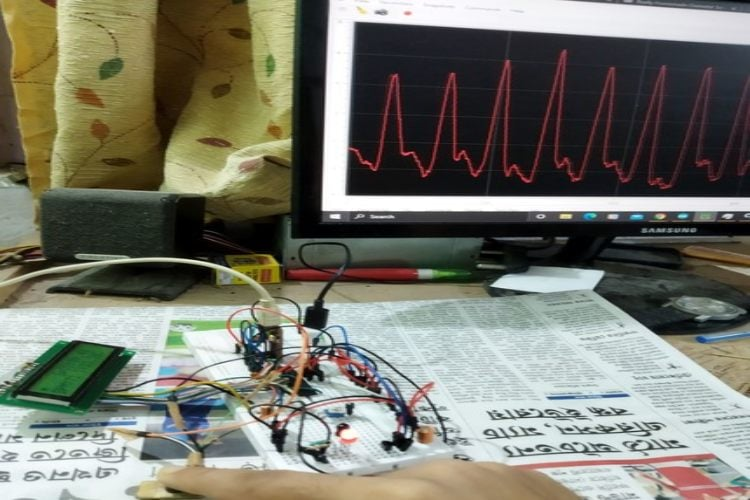789 6
Avijit Das

## Paean A Simple yet Dynamic System for Monitoring of Essential body parameters Like Heart Rate, Oxygen Level and Body Temperature

## Inspiration We all saw how everyone started to panic during the onset of the pandemic, and it...

### Project Used Hardware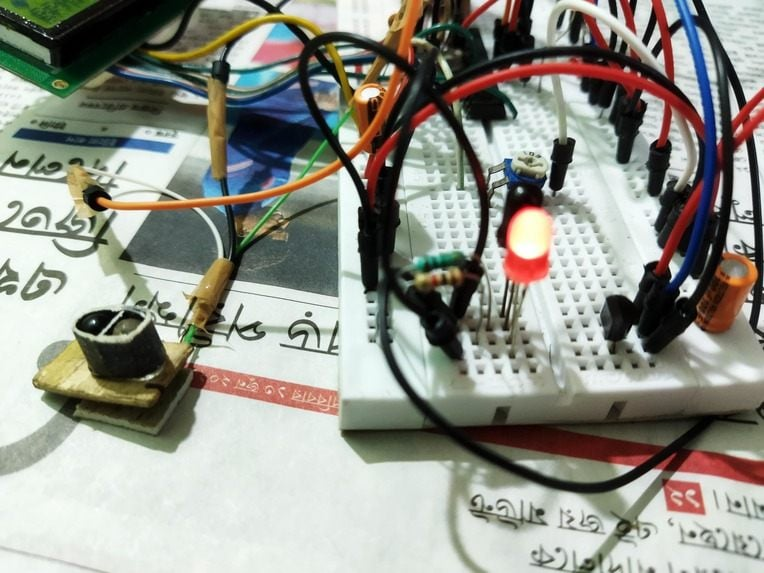AVR microcontroller(atmega328p), IR LED, Photodiode, LM35, Breadboard, wires(a lot of wires), USB to TTL converter(CH340chip)

### Project Used Software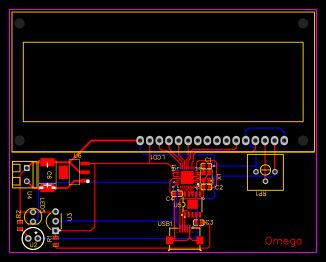Arduino IDE, SerialPlot

### Project Hardware Software Selection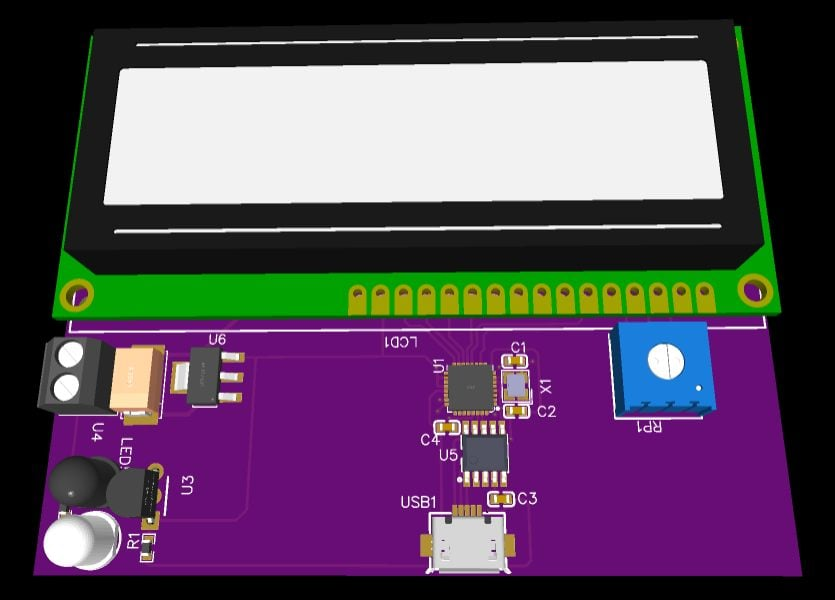### Circuit Diagram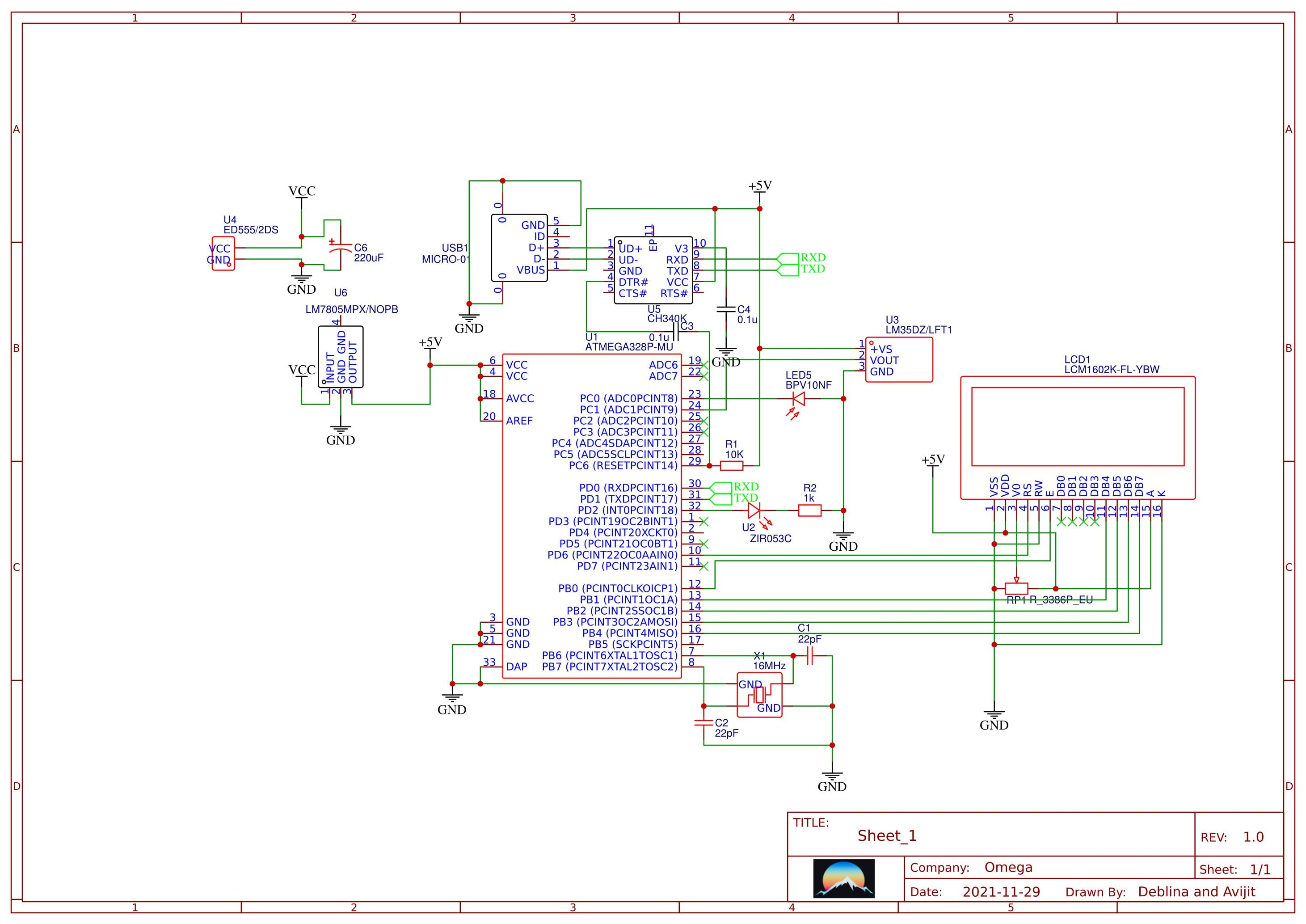The Screw Terminal is given for a battery input source, then it is fed into a 5v voltage regulator that regulates the input voltage to fixed 5v, then there is a 16x2 LCD for showing the measurements, and for the sensor we have the IR LED and Photodiode, along with that a LM35 temperature Sensor. These sensors were interfaced by the microcontroller sitting at the middle and the USB to TTL chip Ch340 sitting below the microcontroller is used to interface it with the computer, and for connecting Paean to the computer a micro USB port is given which can be connected by a USB cable.

##### Code

``` ```

`#include <LiquidCrystal.h>`

``` #define alpha 0.2 #define alpha2 0.05 #define alphar 0.4 #define alphar2 0.002 #define nIR 200 #define nRed 50 int data[nIR]; int dataR[nRed]; byte bheart = {   0b00000,   0b01010,   0b11111,   0b11111,   0b11111,   0b01110,   0b00100,   0b00000 }; byte sheart = {   0b00000,   0b00000,   0b01010,   0b11111,   0b01110,   0b00100,   0b00000,   0b00000 }; const int rs = 9, en = 7, d4 = 6, d5 = 4, d6 = 3, d7 = 2; LiquidCrystal lcd(rs, en, d4, d5, d6, d7); float x, y, raw, sum = 0, avg = 0, braw = 0.0, max = 0, bpm = 0.0, lavg = 0.0, conf, lbpm, spraw=0.0, bpmavg; int idx = 0, idxr=0; int ct = 0; long ls = 0, ps = 0, cs = 0; boolean f=false; float rmin=0,rmax=0, imin=0, imax=0, lspraw=0.0; void setup() {    lcd.begin(16, 2);   lcd.createChar(0, bheart);   lcd.createChar(1, sheart);   lcd.clear();   delay(1000);     lcd.print("     Paean");   lcd.setCursor(1,1);   lcd.print("Place Finger");   float diff=0.0; int f=1; String s=""; ps=millis(); int n=0,c=0; float rawtemp=0, ltemp=0;    pinMode(A0, INPUT_PULLUP);   pinMode(A1, INPUT);     pinMode(A5, INPUT);   Serial.begin(38400);   for(int i=0;i<nIR;i++)   data[i]=0;   for(int i=0;i<nRed;i++)   dataR[i]=0;     delay(3000);   lcd.clear();       lcd.setCursor(0, 1);       lcd.print("BPM:");       lcd.print((int)lavg);          lcd.setCursor(5, 0);       lcd.print("SpO2:");       lcd.print(spraw/10);   for(;;)        {     braw = calRawIR();    rawtemp+=calRawTemp();       if (braw > 2 && f)     {       spraw+=(((diff/rmin)/4.58)*92.26+8.05);       rmax=calRawRed();           ps = millis() - ls;       ls = millis();       f = 0;       n++;         bpmavg += bpm;       bpm = (1000.0 / ps) * 60;       lcd.setCursor(0, 0);       lcd.write(byte(1));              }      else if (braw < -0.5)     {       f = 1;       lcd.setCursor(0, 0);       lcd.write(byte(0));       rmin=calRawRed();       diff=abs(rmin-rmax)*-1;     }    if(n==9)    {     lavg = bpmavg / 10;     lcd.clear();       lcd.setCursor(7, 1);       lcd.print("T:");       lcd.print(ltemp);       lcd.print("C");       lcd.setCursor(0, 1);       lcd.print("BPM:");       lcd.print((int)lavg);       lcd.setCursor(5, 0);       lcd.print("SpO2:");       lcd.print(spraw/10);       rawtemp=0;       spraw=0;       bpmavg=0;     n=0;    }     if(c==1000)     {       c=0;       lcd.setCursor(7, 1);       lcd.print("T:");       if(rawtemp/1000.00>32)       ltemp=rawtemp/1000.00;        lcd.print(ltemp);       lcd.print("C");       rawtemp=0;     }    c++;    Serial.println(braw);   }    } void loop() { } float calRawIR() {   raw = analogRead(A0);   x  = alpha * raw + (1 - alpha) * x;   y  = alpha2 * x + (1 - alpha2) * y;   sum -= data[idx];   data[idx] = raw;   sum += raw;   idx = (idx + 1) % nIR;   avg = sum / nIR;   return (y-avg); } float xr, yr, sumr = 0, avgr = 0, rawr; float calRawRed() {   rawr = analogRead(A1);   xr  = alphar * rawr + (1 - alphar) * xr;   yr  = alphar2 * xr + (1 - alphar2) * yr;   sumr -= dataR[idxr];   dataR[idxr] = (xr-yr)*7;   sumr += (xr-yr)*7;   idxr = (idxr + 1) % nRed;   avgr = sumr / nRed;   return (y-avg)/3.0; } float temp=0.0; float calRawTemp() {     float rawt = analogRead(A5);     temp=0.1*rawt + 0.9*temp     float mv = (temp/870.0)*5002.0;   float cel = mv/10;   return cel; } ```